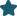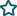How tos

# How To use recursion in Navision?

Author
Alain Krikilion (alias kriki)
Website
http://www.mibuso.com/blogs/kriki
Date
12/12/2005
Size
10,14 KB
2030
RatingPopularity
1. What is recursion?

Recursion is a function/procedure (I’ll use function from now on) that calls itself directly (=in function XYZ, is a reference to function XYZ) or indirectly (in function XYZ is a reference to function ABC in which is a reference to function KLM in …. which is a reference to function XYZ).

2. Skeleton function for recursion

```Function RecursiveFunction(Parameters): Optional RETURN-VALUE
BEGIN
IF {End-Condition} THEN
EXIT(Some Return-Value);

RecursiveFunction(Parameters); // optional, this function can be called more than once

Do something with current variables.

RecursiveFunction(Parameters); // optional, this function can be called more than once
END;
```

• The most important thing about recursion is to know when to stop the recursion.
• Also important is that the variables you use in a recursive function must be local ones. Exception is if something has to be saved globally.
• It is slower then iterative programming, because for recursion, on every procedure-call the local variables, function-information must be saved on the stack and when existing, it must be restored from the stack. However, this is generally not a problem for use in a database-environment, because recursion-performance is expressed in microseconds and DB-access is expressed in milliseconds.
• Recursion has it’s limits in number of recursive calls. This because the computer has a limited amount of stack for it. If you run out of stack, you will get an error.

4. A classic recursion-case

```SUM(N) = 1 + 2 + … + N

Function Sum(Iint : INTEGER): INTEGER
BEGIN
IF Iint = 0 THEN
EXIT(0);
EXIT(Iint + Sum(Iint – 1));
END
```
This function will go Iint levels deep, so the possibility you get out of stack is possible.

The next implementation will avoid it (although not completely), because it will go LOG2(N) (=logarithm with base 2) deep. Try with Sum(50000) for both cases and see the result (stack overflow in the first example and a result in the second).
```Function Sum(Iint : INTEGER) : INTEGER
BEGIN
EXIT(Sum2(0,Iint));
END

Function Sum2(IintFrom : INTEGER;IintTo : INTEGER) : INTEGER
Local variable : Lint: INTEGER // this must be a local variable!
BEGIN
IF IintFrom = IintTo THEN
EXIT(IintFrom);

Lint := (IintTo + IintFrom) DIV 2;

EXIT(sum2(IintFrom,Lint) + sum2(Lint + 1,IintTo));
END;
```

5. In the fob there are 2 reports

• R50000: this shows all the items with their children, and for each child its children, and so on
• R50001 (Luc’s favourite report :) ) : It is possible that a you want to know which components (and how many of each) a parent has. This report goes through the whole BOM-structure and flattens out the components. Basically it makes a single-level BOM of a N-level BOM-structure.